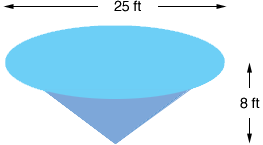Quandaries and Queries HI -   I seem to be having a grey matter moment.  I have a pond that I need to treat with an anti algae solution which needs to applied based on the volume of water in the pond (in gallons).   The rough diameter of the surface of the pond  = 25 ft The depth of the pond = 8 ft The pond shape is conical   How many gallons of water are in my pond?   I'm sure I could have solved this simple equation 35 years ago in when I was in High School,  but somehow ... not today.  I appreciate your assistance in this matter.   Douglas Hi Douglas, I drew a diagram of what I think your pool looks like.The volume of a cone is  1/3r2h where r is the radius of the circular base and h is the height. Your pool has a height of 8 feet and the base of radius 12.5 ft so the volume is  1/3r2h =  1/312.528 = 416.667 cubic feet. I know that there are 231 cubic inches in an American gallon so I need to convert the volume above from cubic feet to cubic inches. There are 12 inches in a foot so there are 123 cubic inches in a cubic foot. Hence the volume of your pool is 416.667123 = 720000 cubic inches. Thus, converting to gallons, the volume is  720000/231 = 3117 gallons. Penny Go to Math Central